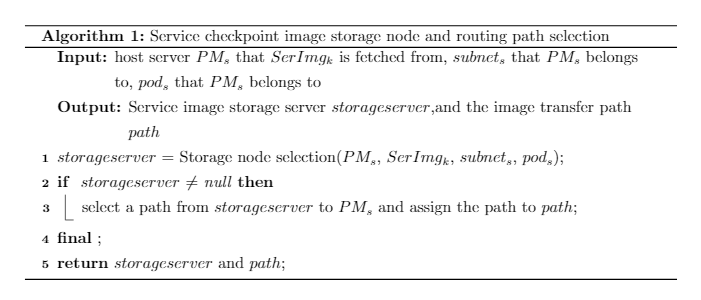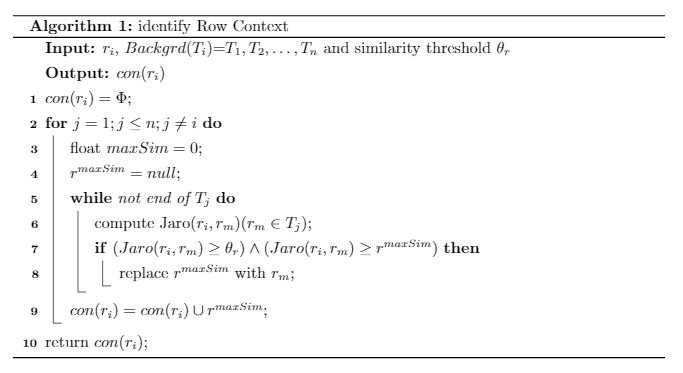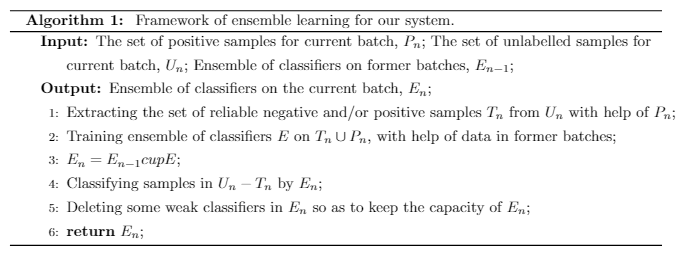• ## 论文撰写——伪代码

万次阅读 多人点赞 2019-10-29 11:46:51
https://blog.csdn.net/taoyafan/article/details/80380651 X：https://jingyan.baidu.com/article/08b6a591969d5a14a8092294.html https://blog.csdn.net/m0_37907383/article/details/90238946 ...
https://blog.csdn.net/taoyafan/article/details/80380651
X：https://jingyan.baidu.com/article/08b6a591969d5a14a8092294.html
https://blog.csdn.net/m0_37907383/article/details/90238946
直接上LaTex：https://www.cnblogs.com/hqium/p/6439312.html
在不能梯子下，解决问题太难了。最后选择在线编辑，固然是一种趋势，毕竟可以放肆地跨平台线上操作。
overleaf在线编辑：https://cn.overleaf.com/project
参考写法：https://blog.csdn.net/golden1314521/article/details/40923377#\documentclass[8pt,twocolumn]{ctexart}
%\usepackage{amssymb}
%\usepackage{bm}
%\usepackage{textcomp} %命令\textacutedbl的包,二阶导符号

% Page length commands go here in the preamble
%\setlength{\oddsidemargin}{-0.25in} % Left margin of 1 in + 0 in = 1 in
\setlength{\textwidth}{12in}   % 指定显示纸张宽度Right margin of 8.5 in - 1 in - 6.5 in = 1 in
%\setlength{\topmargin}{-.75in}  % Top margin of 2 in -0.75 in = 1 in
%\setlength{\textheight}{9.2in}  % Lower margin of 11 in - 9 in - 1 in = 1 in
%\setlength{\parindent}{0in}

% 前期准备——start
\makeatletter
\newif\if@restonecol
\makeatother
\let\algorithm\relax
\let\endalgorithm\relax
\usepackage[linesnumbered,ruled,vlined]{algorithm2e}%[ruled,vlined]{
\usepackage{algpseudocode}
\usepackage{amsmath}
\renewcommand{\algorithmicrequire}{\textbf{Input:}}  % Use Input in the format of Algorithm
\renewcommand{\algorithmicensure}{\textbf{Output:}} % Use Output in the format of Algorithm
% 前期准备——end

\begin{document}

% 主体algorithm部分——start
\begin{algorithm}

\caption{Service checkpoint image storage node and routing path selection}

\LinesNumbered

\KwIn{host server $PM_s$ that $SerImg_k$ is fetched from, $subnet_s$ that $PM_s$ belongs to, $pod_s$ that $PM_s$ belongs to}

\KwOut{Service image storage server $storageserver$,and the image transfer path $path$}

$storageserver$ = Storage node selection($PM_s$, $SerImg_k$, $subnet_s$, $pod_s$)\;

\If{ $storageserver$ $\neq$ null}
{
select a path from $storageserver$ to $PM_s$ and assign the path to $path$\;
}

\textbf{final} \;

\textbf{return} $storageserver$ and $path$;

\end{algorithm}
% 主体algorithm部分——end

\end{document}\documentclass[18pt,twocolumn]{ctexart}
%\usepackage{amssymb}
%\usepackage{bm}
%\usepackage{textcomp} %命令\textacutedbl的包,二阶导符号

% Page length commands go here in the preamble
%\setlength{\oddsidemargin}{-0.25in} % Left margin of 1 in + 0 in = 1 in
\setlength{\textwidth}{12in}   % 纸张宽度Right margin of 8.5 in - 1 in - 6.5 in = 1 in
%\setlength{\topmargin}{-.75in}  % Top margin of 2 in -0.75 in = 1 in
%\setlength{\textheight}{9.2in}  % Lower margin of 11 in - 9 in - 1 in = 1 in
%\setlength{\parindent}{0in}

% 前期准备——start
\makeatletter
\newif\if@restonecol
\makeatother
\let\algorithm\relax
\let\endalgorithm\relax
\usepackage[linesnumbered,ruled,vlined]{algorithm2e}%[ruled,vlined]{
\usepackage{algpseudocode}
\usepackage{amsmath}
\renewcommand{\algorithmicrequire}{\textbf{Input:}}  % Use Input in the format of Algorithm
\renewcommand{\algorithmicensure}{\textbf{Output:}} % Use Output in the format of Algorithm
% 前期准备——end

\begin{document}
\begin{algorithm}
\caption{identify Row Context}
\KwIn{$r_i$, $Backgrd(T_i)$=${T_1,T_2,\ldots ,T_n}$ and similarity threshold $\theta_r$}
\KwOut{$con(r_i)$}
$con(r_i)= \Phi$\;
\For{$j=1;j \le n;j \ne i$}
{
float $maxSim=0$\;
$r^{maxSim}=null$\;
\While{not end of $T_j$}
{
compute Jaro($r_i,r_m$)($r_m\in T_j$)\;
\If{$(Jaro(r_i,r_m) \ge \theta_r)\wedge (Jaro(r_i,r_m)\ge r^{maxSim})$}
{
replace $r^{maxSim}$ with $r_m$\;
}
}
$con(r_i)=con(r_i)\cup {r^{maxSim}}$\;
}
return $con(r_i)$\;
\end{algorithm}
\end{document}\documentclass[8pt,twocolumn]{ctexart}
%\usepackage{amssymb}
%\usepackage{bm}
%\usepackage{textcomp} %命令\textacutedbl的包,二阶导符号

% Page length commands go here in the preamble
%\setlength{\oddsidemargin}{-0.25in} % Left margin of 1 in + 0 in = 1 in
\setlength{\textwidth}{12in}   % 纸张宽度Right margin of 8.5 in - 1 in - 6.5 in = 1 in
%\setlength{\topmargin}{-.75in}  % Top margin of 2 in -0.75 in = 1 in
%\setlength{\textheight}{9.2in}  % Lower margin of 11 in - 9 in - 1 in = 1 in
%\setlength{\parindent}{0in}

% 前期准备——start
\makeatletter
\newif\if@restonecol
\makeatother
\let\algorithm\relax
\let\endalgorithm\relax
\usepackage[linesnumbered,ruled,vlined]{algorithm2e}%[ruled,vlined]{
\usepackage{algpseudocode}
\usepackage{amsmath}
\renewcommand{\algorithmicrequire}{\textbf{Input:}}  % Use Input in the format of Algorithm
\renewcommand{\algorithmicensure}{\textbf{Output:}} % Use Output in the format of Algorithm
% 前期准备——end

\begin{document}
\begin{algorithm}[htb]
\caption{ Framework of ensemble learning for our system.}
\label{alg:Framwork}
\begin{algorithmic}
\Require
The set of positive samples for current batch, $P_n$;
The set of unlabelled samples for current batch, $U_n$;
Ensemble of classifiers on former batches, $E_{n-1}$;

\Ensure
Ensemble of classifiers on the current batch, $E_n$;

\State Extracting the set of reliable negative and/or positive samples $T_n$ from $U_n$ with help of $P_n$;
\label{code:fram:extract}

\State Training ensemble of classifiers $E$ on $T_n \cup P_n$, with help of data in former batches;
\label{code:fram:trainbase}

\State $E_n=E_{n-1}cup E$;

\State Classifying samples in $U_n-T_n$ by $E_n$;
\label{code:fram:classify}

\State Deleting some weak classifiers in $E_n$ so as to keep the capacity of $E_n$;
\label{code:fram:select} \\

\Return $E_n$;
\end{algorithmic}
\end{algorithm}
\end{document}

展开全文• 请大家告诉我: 发表计算机类论文时，伪代码编写，应该遵从什么样的语法、句法规范以及格式规范？人工智能
• Latex用不明白 但是有online的类Latex工具可以使用网址： https://www.overleaf.com/
Latex用不明白
但是有online的类Latex工具可以使用网址：
https://www.overleaf.com/
展开全文• 写论文时候需要用伪代码描述算法，记录下，以后用到就来看一看。 循环 for each ρ∈T\rho \in Tρ∈T do  … while Worklist≠∅Worklist \ne \emptyWorklist​=∅ do  … 无论是for循环还是while循环大致都...
写论文时候需要用伪代码描述算法，记录下，以后用到就来看一看。 写循环 for each

ρ

∈

T

\rho \in T

do  … while

W

o

r

k

l

i

s

t

≠

∅

Worklist \ne \empty

do  … 无论是for循环还是while循环大致都是一样的。 伪代码就是：能够清楚表达算法过程即可。大白话的描述也是伪代码，只是写在论文中，就。。。等着退稿吧。 看了一些别人的算法，伪代码一般使用斜体。关键字加粗。大体框架是 function

N

A

M

E

N_{AME}

input   arg - 参数描述 begin  算法正文 /*注释*/ end 注释使用块注释，我当时手贱，使用了python#注释，被批评了。 算法名字使用英文全大写。第一个字母大一点，后面小一点。我不知道这是怎么处理，我看英文期刊给的模板就是这样的。我的方式是直接套用IEEE给的模板。如果期刊要求不严格，可以随意。
展开全文• 这里主要是想说一下如何在word中插入算法的伪代码。 一、使用Aurora插件 二、使用表格
写在前面
这里主要是作为补充，想说一下如何在word中插入算法的伪代码。
关于word的其他论文排版技巧，可以参见之前的word论文排版和写作01：样式、自动列表、图片、表格、公式、脚注、目录、页眉页脚及各种交叉引用一文。
一、使用Aurora插件
如果是要插入英文的伪代码，可以使用Aurora插件，直接参考博客：如何在Word中优雅地插入伪代码，里面说得很详细了。 Aurora下载的地址为：https://xiazai.xiazaiba.com/Soft/A/aurora-setup_XiaZaiBa.zip。
1.使用Aurora的好处是：
使用Latex语言编码，在格式生成方面很强大，也方便管理。可以直接作为word的对象插入，插入后类似于图片的效果。
2.使用Aurora的缺点是：
对生成中文的伪代码极不友好。这应该是使用Latex的通病了，就是对中文的支持远不如英文那么方便。调配过程门槛较高，出BUG的机会较大，随心所欲地调整格式难度较高。毕竟是类似Latex编程的，肯定远没有word的“所见即所得”用起来得心应手。而且这个插件后续已经很久没有维护了，所以使用起来肯定比较难受。
3.安装和使用方式：
安装就直接解压安装就行。注意，安装后需要重启word，然后word就能直接将Aurora包含到对象中。点击插入，选择文本中的对象，然后在对象类型中选择Aurora Equation。此时将在文中出现一个正方形框，同时打开Aurora的编辑界面。点击编辑界面的Properties调出Preferences设置框，其中，在Properties下可以设置字体的大小，分辨率和区域的宽度。注意调整区域宽度，该值应该等于页面宽度减去2倍的页边距。否则公式（也就是伪代码的区域）会超过页边距。在Packages中加入要用到的包。然后在编辑界面的空白区域编写算法的伪代码。最后点击左上角的Refresh按钮，即可在文中显示生成的伪代码。
二、使用表格
诚然，Aurora虽然类似Latex，但是就是过于麻烦了，而且使用插件总有一种不太安心的感觉。有没有什么word原始方式进行伪代码的编写呢？当然有了，这个方法就是使用插入表格（怎么又是表格~~）。
1.使用插入表格的方式的优点有：
不用安装什么插件，学习什么Latex语法，还有debug。格式调整方便，所见即所得。对中英文的伪代码插入均同等方便。这个简直秒杀Aurora。支持所有word自带的功能，包括插入题注和交叉引用等。
2.唯一的缺点是：
格式调整起来比较麻烦，不像Latex那样有一个统一的模板，需要自己重新设置。
这里好像有些前后矛盾了，在优点里面说它格式调整方便，但是缺点里面又说格式调整麻烦。其实不是的。前面的方便是指在表格中设置格式是方便的，你几乎能够实现所有word中能够设置的所有格式，包括字体的所有设置，行距的所有设置，都可以自己调整，这在Aurora中就比较复杂了，还得去查对应的编码和导入对应的包，还不一定能够实现。后面的麻烦是指它没有模板，所以什么都要自己调，不像Aurora那样直接就可以开始写了，能够套模板。
3.使用方法：
插入一个三行一列的表格选中表格，然后选择引用->插入题注，新建一个算法的题注，然后确定。算法的题注一般是在表格的第一行的，但word限制了只能在表格的上方或者下方插入题注，所以需要将插入后的题注剪切到第一行中，然后按照要求修改题注的格式。第二行写输入和输出的变量。第三行是具体的伪代码。每一行代码前面需要序号，可以用word的自动编号。代码的缩进量为4个空格，注意不能用Tab键，这里是会跳转到下一格而不会插入制表符。其余部分的编写完全按照正文的编写方法，可以插入公式，也可以插入引用。修改行距为固定的20磅。注意，修改后如果某些公式没有显示完全（因为固定行距会忽略图片或者对象的实际大小，不会自适应调整），需要单独修改该行为单倍行距。最后将左右边框和第二格与第三格之间的内边框隐藏即可。
效果类似下图（不是我做的，是一篇论文的截图），但是是没有竖线的啦，并不知道如何弄竖线；另外，每行代码序号的自动编码的格式可以自定义，把句号去掉做成下面的效果：展开全文doc
• Aurora安装和使用 LaTex 入门语法 LaTex数学公式和符号
• 1.sfc-v2-3 这个流程图工具可以直接输出伪代码,画程序逻辑最好 sfc-v2-3.exe 是我汉化的版本 eXeBat.exe 是汉化工具(发现汉化不完全的地方可以继续汉化) 基本够用了 2.玲珑流程图 是一个简易的基本流程图工具 以上...
• 该篇文章讲述了论文实验部分的伪代码，该实验采用python语言编写，框架采用深度学习框架keras，整体实验分为一下几个部分： 1 第一次训练（first.py） 功能实现： 根据输入的数据文件，处理数据后，切割为训练集和...
• ## 伪代码书写规则

万次阅读 多人点赞 2019-06-20 10:54:51
最近要用到伪代码写算法，所以在网上查阅了一些资料，写这篇博客正好整理、记录一下自己所学的书写规则，以便自己日后使用，如果能对大家有所帮助，那就更好了。文中不足，欢迎给位大神多多指点。 输入 赋值语句用...程序设计 语法规则 循环结构 注释
• ## Word中伪代码编写

千次阅读 2020-02-25 20:35:03
伪代码的写法 伪代码规范 Latex算法的伪代码排版
• WPS中用Aurora写伪代码 第一步 在Properties->Packages中添加 \usepackage{algorithm} \usepackage{algpseudocode} \usepackage{amsmath} \usepackage{setspace} \renewcommand{\algorithmicrequire}{\textbf{...LaTeX Aurora
• 示例： \documentclass[11pt,twoside,a4paper]{article} \usepackage{algorithm} \usepackage{algorithmicx} \usepackage{algpseudocode} \usepackage{amsmath} ...%% 算法伪代码或者流程的前期准备 \renewc
• 关于Latex的安装，这里我用到了Texstudio，大家戳下面的链接按照步骤进行下载：CSDN：最简单傻瓜式的 Latex+Texstudio 安装教学...在Texstudio/winEdit或者直接 http://overleaf.com网页版进行...
• 论文原始图片 程序说明,解释//对象x的CRUSH放置算 procedure TAKE(a) // Put item a in working vector~i { ~i←[a] }//end procedure procedure SELECT(n,t) // Select n items of type t { ~o←0 //输出结果o,...
• 实验报告遇到的，有个链接真的好，适合我这种会一丢丢latex的人快速上手。latex算法的伪代码排版
• 之前自己尝试用visio+mathtype画算法流程图，但是总是存在着各种各样的问题，今天才知道最好的算法流程图是用latex出来的，在网上找到相关资源，先记录下来，然后照着实践一下看看效果。 转载自： ...
• 文章目录基本组成部分变量的声明表达式赋值语句指令的表示注释函数特殊结构分支...对于一些子任务，可以一句话带过，避免与主题无关的细节扰乱了代码整体； 比如：“假设x是列表A中最小的项”、“从列表A中删除x”。
• ## latex 写算法伪代码

千次阅读 2020-08-05 21:33:38
最近写论文要在latex里写伪代码，网上的教程几乎都用不了。要么就是说没有安装相关包，一些对我无用的安装/导入教程，要么就是直接贴了很多看似有用其实跑不通的代码。 之前在电脑上安装了WinEdt，后来投入了...latex
• 1. for,end for,do,while, end while,if,else都用加粗 2. 变量要用花体 3. 不要使用括号，而是使用缩进表示代码块 4. 需要有input，output 5. 需要有行标
• 准备工作： 1、先安装 CTEXhttps://mirrors.tuna.tsinghua.edu.cn/ctex/legacy/2.9/ 2、下载algorithm2e 包（里面有详细的使用说明文档）... 这个其实 CTEX已经包含了，不过不是最...
• 有在word中写伪代码的需求，公式倒可以用MathType替代，但是伪代码好像比较麻烦，因此搜索到了可以使用Aurora插件实现。 实际安装使用过程中发现，网上的很多资料要么就是不全面，有错误，要么就是没有详细到能够...Word Aurora
• 2....}} \renewcommand{\algorithmicensure}{\textbf{Output:... 到这里，就结束了，剩下的工作就是学会使用这种语法，将自己的伪代码放到论文中去！ 如果百度网盘链接失效（我设置了永久有效）或者哪里有问题可以留言。
• dfs 伪代码 dfs(...) //含义：（！！）准备 对当面步 做决定 { if(ok==1) return; if(到终点) { ok=1； ... return; } ... do //有时 当前步只有一个决定 { （1）对当前步 做第一个...
• ## 伪代码书写规范

万次阅读 2019-03-20 16:48:37
伪代码（Pseudocode）是一种算法描述语言。使用伪代码的目的是为了使被描述的算法可以容易地以任何一种编程语言（Pascal，C，Java，etc）实现。因此，伪代码必须结构清晰、代码简单、可读性好，并且类似自然语言。 ...
• 伪代码（Pseudocode）是一种算法描述语言。使用伪代码的目的是为了使被描述的算法可以容易地以任何一种编程语言（Pascal，C，Java，etc）实现。因此，伪代码必须结构清晰、代码简单、可读性好，并且类似自然语言。 ...
• 哪位同学知道怎么用overleaf插入到word请赐教，毕竟在线编辑还是最方便的嘛（懒汉的心声哈哈） 注2：前些年的网上代码大多不可用，大家不用费劲调试了。 挖个坑 争取月底填 终于准备一些东西了，不准备做技术向博...
• matlab代码如何改成伪代码基于伪序列的光场图像压缩 我们在这里提供我们论文的源代码： Dong Liu, Lizhi Wang, Li Li, Zhiwei Xiong, Feng Wu, Wenjun Zeng: 基于伪序列的光场图像压缩。 2016 IEEE 国际多媒体会议和......